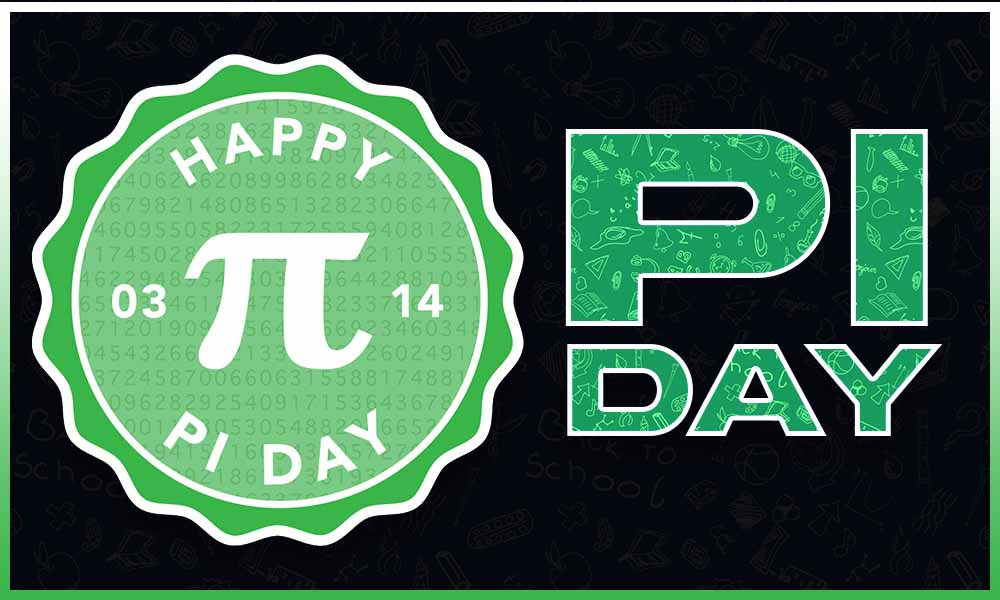# What is Pi Day?Prelims: Current Affairs & Events### Overview:

#### Pi Day was recently celebrated worldwide to recognize the mathematical constant, Pi.

• International Day of Mathematics, or Pi Day, is celebrated on March 14 every year to recognize the mathematical constant, Pi.
• The day is celebrated by mathematics enthusiasts and educators worldwide to recognize and appreciate the significance of mathematics in our lives.
• Why March 14?
• The approximate value of Pi is 3.14.
• The date, when written in the format of month/day (3/14), matches the first three digits of the mathematical constant.
• History:
• The first Pi Day was celebrated in 1988 by physicist Larry Shaw at the San Francisco Exploratorium.
• In 2009, the United States House of Representatives designated March 14 as Pi Day.
• UNESCO marked Pi Day as the ‘International Day of Mathematics' during its general conference in 2019.
• Mathematician and physicist Albert Einstein, known for the "General Theory Of Relativity," was born on Pi Day in 1879.

### What is Pi (π)?

• Pi is a mathematical constant that represents the ratio of the circumference of a circle to its diameter.
• It is an infinitely long, irrational number, and its exact value cannot be known.
• Pi is roughly equal to 3.14 or 22/7.
• History:
• Pi has been known for nearly 4,000 years and was discovered by the ancient Babylonians.
• One of the first calculations of pi was carried out by Greek mathematician Archimedes of Syracuse (287 B.C. to 212 B.C.)
• It also has a symbol, ‘π.’ The symbol π was devised by British mathematician William Jones in 1706.
• Applications: It is used in a wide variety of mathematical and scientific calculations, including geometry, trigonometry, calculus, and more.

### Q1) What is an irrational number?

An irrational number is any real number that cannot be expressed as the quotient of two integers—that is, p/q, where p and q are both integers. Irrational numbers such as π can be expressed as an infinite decimal expansion with no regularly repeating digit or group of digits. Together the irrational and rational numbers form the real numbers.